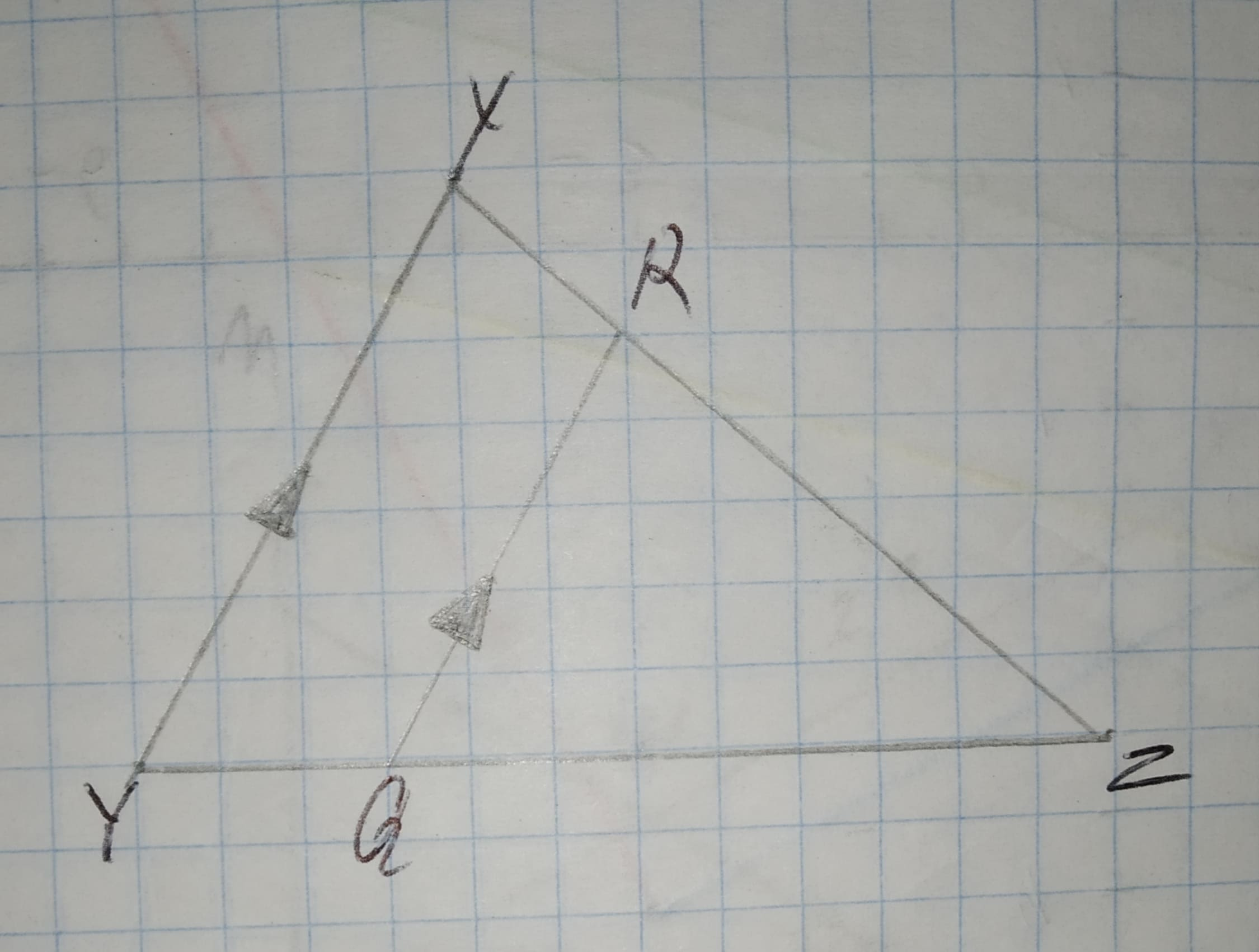Question# Whether the triangle are similar. if so write the similarity statement and if not what would be the sufficient to prove the triangle similar.Given:12210203141.jpg

Similarity
ANSWEREDWhether the triangle are similar. if so write the similarity statement and if not what would be the sufficient to prove the triangle similar.
Given:2020-12-13
Conclusion:
In triangle
RQZ and XYZ
$$\displaystyle\angle{Z}=\angle{Z}$$ (common)
$$\displaystyle{R}{Q}{\mid}{\mid}{X}{Y}$$
therefore
$$\displaystyle\angle{Z}{Q}{R}=\angle{Z}{Y}{X}$$ (corresponding)
thus
$$\displaystyle\triangle{R}{Q}{Z}\sim\triangle{X}{Y}{Z}$$
by AA similarity.
Thus
The triangle are similar.